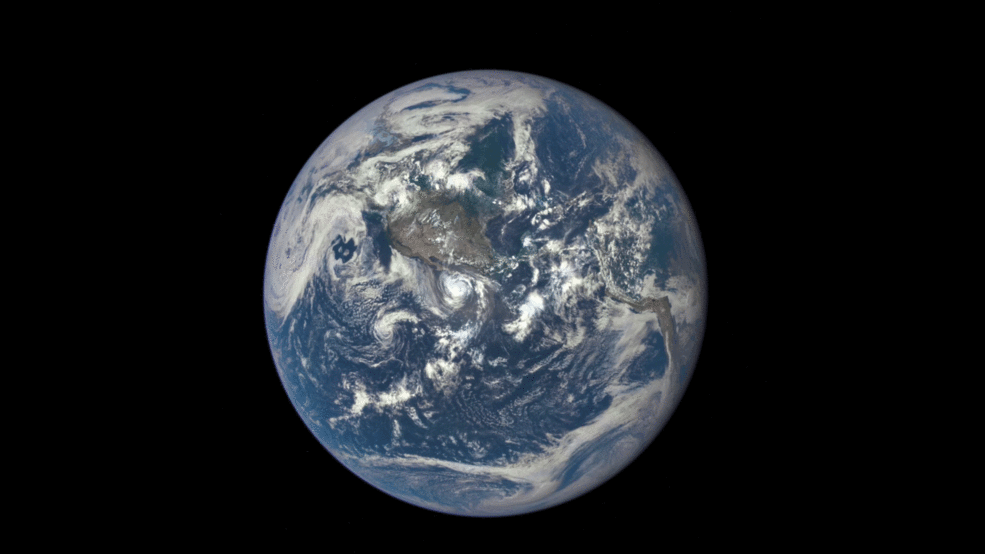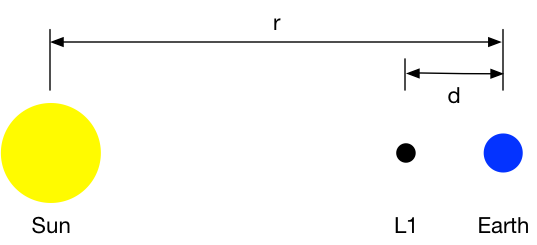# Satellite of love

You may have seen this animated GIF a day or two ago. It’s from NASA and was put together from a series of photos taken by the DSCOVR satellite as the Moon crossed the face of the Earth—what astronomers call a transit.Source: NASA.

If the face of the Moon looks unfamiliar to you, it’s because you’re seeing the far side, the side that always points away from the Earth.

In the article, NASA says the camera on DSCOVR

maintains a constant view of the fully illuminated Earth as it rotates, providing scientific observations of ozone, vegetation, cloud height and aerosols in the atmosphere. Once EPIC [the camera] begins regular observations next month, the camera will provide a series of Earth images allowing study of daily variations over the entire globe. About twice a year the camera will capture the moon and Earth together as the orbit of DSCOVR crosses the orbital plane of the moon.

How does a satellite get “a constant view of the fully illuminated Earth”? It has to maintain an orbit about the Sun that keeps it continuously between the Earth and the Sun. As a general rule, Kepler’s Laws don’t allow this. The closer an object is to the Sun the faster it revolves around it. This is why Mercury’s year is 88 Earth days long and Venus’s is 225 Earth days long. But there is a special spot between the Earth and the Sun where a satellite can stay on a line between the two, seemingly violating the laws of orbital mechanics.

The spot was discovered by Joseph-Louis Lagrange, and it, along with four other spots in which a satellite can maintain an orbit in lockstep with the Earth, are known as the Lagrange points. DSCOVR is at the first Lagrange point, known as L1.

Lagrange is a name you’ll become very familiar with if you do any work in mechanics. He was a 18th century mathematician/astronomer/whatever who took Newton’s mechanics, which was based on forces, and reformulated it in terms of potential and kinetic energy. I spent one semester in grad school where it seemed like all I did was generate differential equations of vibrating systems using Lagrange’s equations.

The Lagrange points are special solutions to the three-body problem in orbital mechanics and the position of L1 is actually fairly easy to derive (once you know it’s there). The NASA and NOAA articles say it’s a million miles away from the Earth, on a line between the Earth and the Sun. Let’s confirm that.

Here’s the layout, obviously not to scale.We’ll call the mass of the Sun $$M$$, the mass of the Earth $$m$$, and the mass of the satellite $$\tilde{m}$$. We’ll find that we don’t need to know the values of any of these, but we will need to know the ratio of Earth and Sun masses, which we’ll call $$\mu$$:

$\frac{m}{M} = \mu = 3\times10^{-6}$

The gravitational force that the Sun exerts on the Earth is, by Newton’s second law, equal to the mass of the Earth times the centripetal acceleration of the Earth as it travels in its nearly circular orbit:

$F = \frac{G M m}{r^2} = m \frac{v^2}{r} = m a$

where $$v$$ is the velocity of the Earth. The velocity of the Earth is related to its period (or year) by recognizing that the distance traveled in one period is the circumference of the orbital circle:

$v T = 2 \pi r$

After some algebra, these two equations can be turned into the single relation:

$T^2 = \frac{4 \pi^2}{G M} r^3$

This matches up with Kepler’s third law, which states that the square of the period is proportional to the cube of the semimajor axis. Because we’re assuming a circular orbit, the semimajor axis is the radius.

Let’s do the same analysis for the satellite. In this case, there are two forces acting on the body: an inward pull from the Sun and an outward pull from the Earth.

$\frac{G M \tilde{m}}{(r-d)^2} - \frac{G m \tilde{m}}{d^2} = \tilde{m} \frac{\tilde{v}^2}{r-d}$

The velocity/period relationship for the satellite is

$\tilde{v} T = 2 \pi (r-d)$

Combining these, we get a somewhat more complicated expression for the square of the period:

$T^2 = \frac{4 \pi^2}{G \left[ M - m \left( \frac{r-d}{d} \right)^2 \right]} (r-d)^3$

Why doesn’t this fit in with Kepler’s third law? Because Kepler’s laws are for two-body problems and we’re looking at a three-body problem.

Because the period of our satellite at L1 is supposed to have the same period as the Earth, we can set the right-hand sides of the two equations for $$T^2$$ equal to one another:

$\frac{4 \pi^2}{G M} r^3 = \frac{4 \pi^2}{G \left[ M - m \left( \frac{r-d}{d} \right)^2 \right]} (r-d)^3$

This is a mess. We can make it much simpler if we introduce a new variable to represent the ratio of $$d$$ and $$r$$:

$\frac{d}{r} = \delta$

After a bit of algebra, we get this:

$(1 - \delta)^3 = 1 - \mu \left( \frac{1}{\delta} - 1\right)^2$

We know the value of $$\mu$$, so the only thing to solve for is $$\delta$$. Because $$\mu$$ is a very small number, studying the equation should lead us to expect $$\delta$$ to be relatively small, too. If that’s true, then we can say

$(1 - \delta)^3 \approx 1 - 3\delta$

and

$\left( \frac{1}{\delta} - 1\right)^2 \approx \frac{1}{\delta^2}$

which turns our equation into

$1 - 3\delta = 1 - \mu \frac{1}{\delta^2}$

The solution to that is

$3\delta^3 = \mu = 3\times10^{-6}$

or

$\delta = 0.01$

If we were to do the full nonlinear solution (without using the small-number approximations), the solution is

$\delta = 0.00997$

so the approximation is pretty damned good, certainly good enough for the precision we’re aiming at here.

So if $$\delta = 0.01$$, then $$d = 0.01 r$$. And because the Earth is 93 million miles from the Sun, that means

$d = 930,000\,\mathrm{miles}$

For the purposes of a popular article, both NASA and NOAA rounded this to a million miles. Because the Moon’s orbital radius is about a quarter of a million miles, that puts DSCOVR in a great spot to take photos of the Moon’s transit of the Earth.

Although the L1 position represents an orbit in lockstep with the Earth’s, it isn’t a stable position. What that means is that if a satellite drifts a bit from L1 (as will happen when it gets pulled by the Moon), it won’t tend to return. So DSCOVR, and the other satellites at L1, need corrections to get back in place.

Thanks to Craig Hockenberry, whose tweet about the GIF led me to NASA’s article. Don’t blame him for the math.